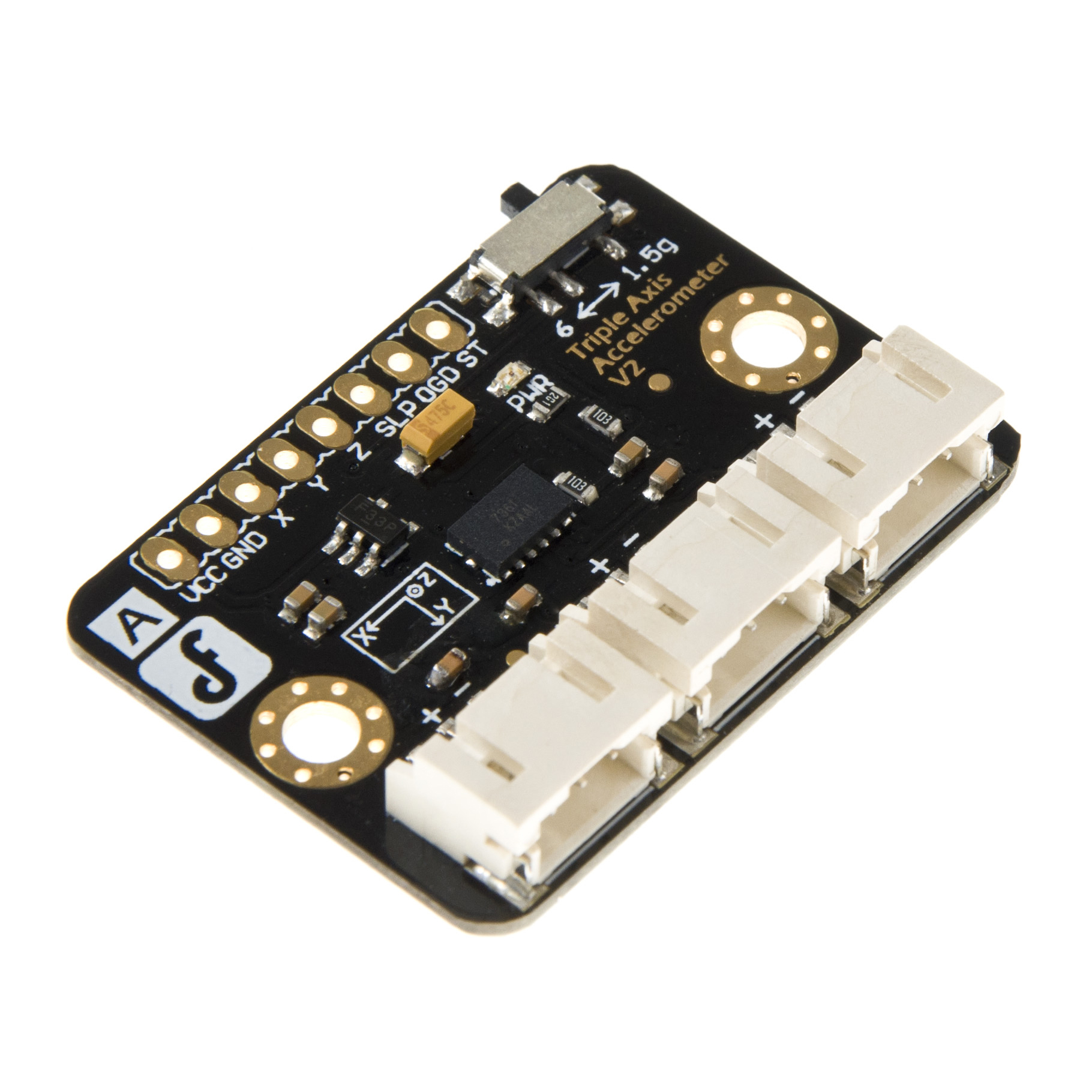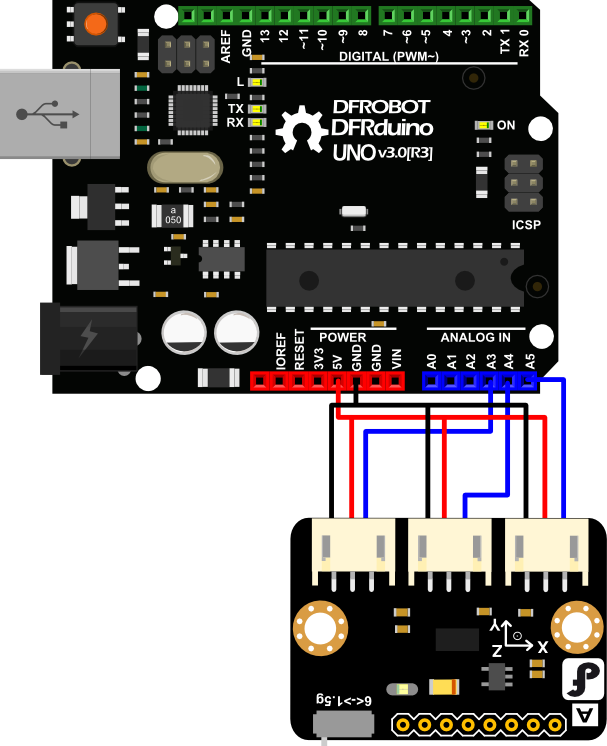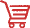# Triple_Axis_Accelerometer_MMA7361_SKU_DFR0143-DFRobot## Introduction

The MMA7361 from Freescale is a very nice sensor with easy analog interface. The MMA7361 is a 3.3V part and outputs an analog voltage for each of the three outputs. This voltage is in ratio to the measured acceleration and to the supply voltage (ratiometric). It has selectable sensitivity by dip switch. You will need some extra hardware to convert this analog signal to a usable digital one. The Arduino is really good option for it. This break board is especially designed for Arduino which has 3 JST connector that can be easily plug into our IO/Sensor expansion board.

## Applications

• Measure the acceleration information for your robot or other devices
• Using for slope alarm or other kinds of alarm such as shock

And we have collected some useful 3-axis data processing methods: How to Use a Three-Axis Accelerometer for Tilt Sensing.

## Specification

• Voltage:3.3-8V
• Selectable sensitivity:±1．5g/6g
• Low power:500μA @ measurement mode，3μA @standby ；
• High sensivity: 800 mV/g @ 1.5g；
• Interface:Analog Output
• Low pass filter
• Size:37x26mm

## Connection Diagram## Sample Code

For Arduino

``````
// # Editor    :Holiday from DFRobot
// # Data      09.10.2013

// # Product name:Triple Axis Accelerometer MMA7361
// # Product SKU:SEN0143
// # Version :  2.0

// # Description:
// # This sample shows how to measure the angle value using two axis value

#include<math.h>
#include<stdio.h>

#define A_X  5
#define A_Y  4
#define A_Z  3

int val_x,val_y,val_z;
double b;
void setup()
{
pinMode(A_X,INPUT);
pinMode(A_Y,INPUT);
pinMode(A_Z,INPUT);
Serial.begin(9600);
}
void loop()
{
float a;
for (int i=0;i<10;i++)
{
}
val_x=val_x/10;
val_y=val_y/10;
val_z=val_z/10;
delay(300);
Serial.print(" X_Axis: ");
Serial.print(val_x);
Serial.print(" Z_Axis: ");
Serial.print(val_z);
Serial.print("      ");
b=(double) (abs(val_x-320))/(abs(val_z-320));
Serial.print(" B: ");
Serial.print(b);
Serial.print("      ");
a=atan(b);
Serial.print(" A: ");
Serial.println(a/3.14*180);              //the value of Angle
val_x=0;
val_y=0;
val_z=0;

}``````

For Micropython

``````from machine import ADC,Pin
import timeGet Triple Axis Accelerometer MMA7361_DFR0143 from DFRobot Store or DFRobot Distributor.